Paul's Online Notes
Home / Calculus I / Extras / Area and Volume Formulas
Show Mobile Notice Show All Notes Hide All Notes
Mobile Notice
You appear to be on a device with a "narrow" screen width (i.e. you are probably on a mobile phone). Due to the nature of the mathematics on this site it is best views in landscape mode. If your device is not in landscape mode many of the equations will run off the side of your device (should be able to scroll to see them) and some of the menu items will be cut off due to the narrow screen width.

### Appendix A.6 : Area and Volume Formulas

In this section we will derive the formulas used to get the area between two curves and the volume of a solid of revolution.

#### Area Between Two Curves

We will start with the formula for determining the area between $$y = f\left( x \right)$$ and $$y = g\left( x \right)$$ on the interval $$\left[ {a,b} \right]$$. We will also assume that $$f\left( x \right) \ge g\left( x \right)$$ on $$\left[ {a,b} \right]$$.

We will now proceed much as we did when we looked that the Area Problem in the Integrals Chapter. We will first divide up the interval into $$n$$ equal subintervals each with length,

$\Delta x = \frac{{b - a}}{n}$

Next, pick a point in each subinterval, $$x_i^*$$, and we can then use rectangles on each interval as follows.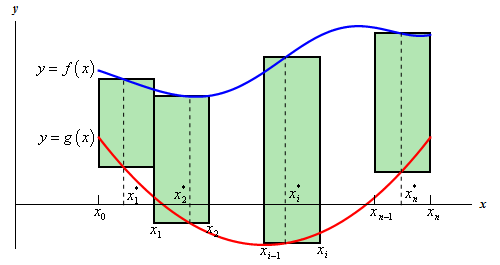The height of each of these rectangles is given by,

$f\left( {x_i^*} \right) - g\left( {x_i^*} \right)$

and the area of each rectangle is then,

$\left( {f\left( {x_i^*} \right) - g\left( {x_i^*} \right)} \right)\Delta x$

So, the area between the two curves is then approximated by,

$A \approx \sum\limits_{i = 1}^n {\left( {f\left( {x_i^*} \right) - g\left( {x_i^*} \right)} \right)\Delta x}$

The exact area is,

$A = \mathop {\lim }\limits_{n \to \infty } \sum\limits_{i = 1}^n {\left( {f\left( {x_i^*} \right) - g\left( {x_i^*} \right)} \right)\Delta x}$

Now, recalling the definition of the definite integral this is nothing more than,

$A = \int_{{\,a}}^{{\,b}}{{f\left( x \right) - g\left( x \right)\,dx}}$

The formula above will work provided the two functions are in the form $$y = f\left( x \right)$$ and $$y = g\left( x \right)$$. However, not all functions are in that form.

Sometimes we will be forced to work with functions in the form between $$x = f\left( y \right)$$ and $$x = g\left( y \right)$$ on the interval $$\left[ {c,d} \right]$$ (an interval of $$y$$ values…). When this happens, the derivation is identical. First we will start by assuming that $$f\left( y \right) \ge g\left( y \right)$$ on $$\left[ {c,d} \right]$$. We can then divide up the interval into equal subintervals and build rectangles on each of these intervals. Here is a sketch of this situation.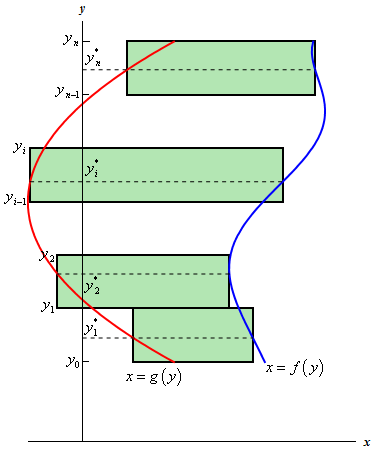Following the work from above, we will arrive at the following for the area,

$A = \int_{{\,c}}^{{\,d}}{{f\left( y \right) - g\left( y \right)\,dy}}$

So, regardless of the form that the functions are in we use basically the same formula.

#### Volumes for Solid of Revolution

Before deriving the formula for this we should probably first define just what a solid of revolution is. To get a solid of revolution we start out with a function, $$y = f\left( x \right)$$, on an interval $$\left[ {a,b} \right]$$.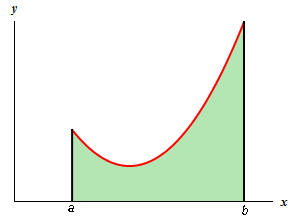We then rotate this curve about a given axis to get the surface of the solid of revolution. For purposes of this derivation let’s rotate the curve about the $$x$$-axis. Doing this gives the following three dimensional region.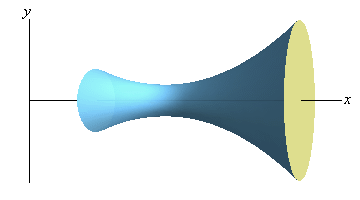We want to determine the volume of the interior of this object. To do this we will proceed much as we did for the area between two curves case. We will first divide up the interval into $$n$$ subintervals of width,

$\Delta x = \frac{{b - a}}{n}$

We will then choose a point from each subinterval, $$x_i^*$$.

Now, in the area between two curves case we approximated the area using rectangles on each subinterval. For volumes we will use disks on each subinterval to approximate the area. The area of the face of each disk is given by $$A\left( {x_i^*} \right)$$ and the volume of each disk is

${V_i} = A\left( {x_i^*} \right)\Delta x$

Here is a sketch of this,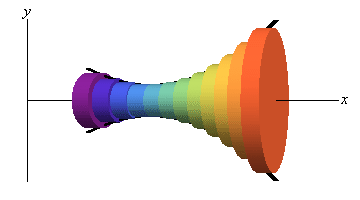The volume of the region can then be approximated by,

$V \approx \sum\limits_{i = 1}^n {A\left( {x_i^*} \right)\Delta x}$

The exact volume is then,

\begin{align*}V & = \mathop {\lim }\limits_{n \to \infty } \sum\limits_{i = 1}^n {A\left( {x_i^*} \right)\Delta x} \\ & = \int_{{\,a}}^{{\,b}}{{A\left( x \right)\,dx}}\end{align*}

So, in this case the volume will be the integral of the cross-sectional area at any $$x$$, $$A\left( x \right)$$. Note as well that, in this case, the cross-sectional area is a circle and we could go farther and get a formula for that as well. However, the formula above is more general and will work for any way of getting a cross section so we will leave it like it is.

In the sections where we actually use this formula we will also see that there are ways of generating the cross section that will actually give a cross-sectional area that is a function of $$y$$ instead of $$x$$. In these cases the formula will be,

$V = \int_{{\,c}}^{{\,d}}{{A\left( y \right)\,dy}},\,\,\,\hspace{0.25in}c \le y \le d$

In this case we looked at rotating a curve about the $$x$$-axis, however, we could have just as easily rotated the curve about the $$y$$-axis. In fact, we could rotate the curve about any vertical or horizontal axis and in all of these, case we can use one or both of the following formulas.

$V = \int_{{\,a}}^{{\,b}}{{A\left( x \right)\,dx}}\hspace{0.25in}\hspace{0.25in}\hspace{0.25in}V = \int_{{\,c}}^{{\,d}}{{A\left( y \right)\,dy}}$